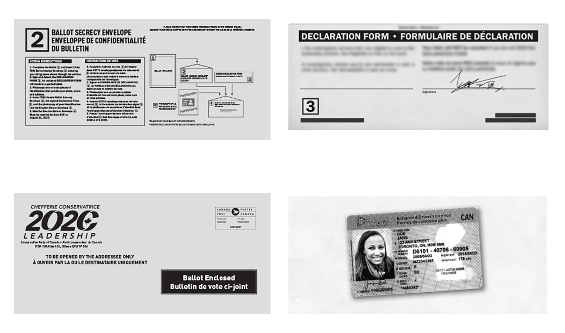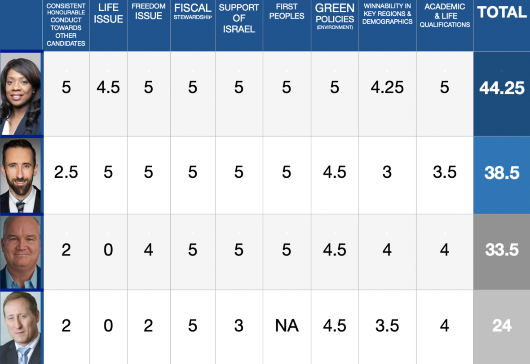Like   Tweet   Pin   +1   in/* styles */ August 10th, 2020

This is very likely, the last week for you to mail in your ballot for the CPC race and have it arrive on time for it to be counted by the August 21st deadline. Even at that, it may not arrive if you simply send it regular mail.

## Drop Locations & Ballot Pick Up Tours With Candidates:

But we have good news! Campaigns have drop locations & candidates are doing ballot pick up tours. (See below for tour spots.)

Click here to see drop-off spots arranged by the Lewis Campaign Team.

## TOUR STOPS IN THE NEXT DAYS

 table div table+table+table+table+table div table{width:100%;padding:0}table div table+table+table+table+table div table img{width:96.23%;padding:0;float:none}table div table+table+table+table+table div table td{width:100%;padding:0 1.88% 18px}/* styles */table div table+table+table+table+table+table div table td,table.module-5{width:100%;padding:0}table div table+table+table+table+table+table div table{width:100%;float:none;margin-left:auto;margin-right:auto;padding:0}table div table+table+table+table+table+table div table a{border:0 none;text-decoration:none}table div table+table+table+table+table+table div table img{width:100%!important;border:0 none;text-decoration:none}/* styles */

# DR. LESLYN LEWIS MAY BE COMING TO YOUR AREA

Dr. Leslyn Lewis will be visiting Saskatchewan and Alberta in the next few days, if you would like to meet her in person and drop off your ballot so it can be delivered securely.

## Today she will be in:

Yorkton

Today - 11am, 12:00pm and 1:00pm MDT - Prairie Harvest Christian Centre, 72 Melrose Ave, Yorkton - Click here to RSVP

Regina

Today - 7pm, 7:45pm and 8:30pm MDT - Lighthouse to All Nations, 686 Pasqua St., Regina - Click here to RSVP

## Tomorrow - Tuesday, August 11, she will be in:

Tomorrow - Tuesday August 11th - Noon, 12:45pm, 1:30pm MDT - Saskatoon Inn Courtyard Room - Click here to RSVP

North Battleford

Tomorrow - Tuesday August 11th - 3:30pm MDT, Tim Horton's Parking Lot, 92 Battleford Place - Click here to RSVP

Lloydminster

Tomorrow - Tuesday August 11th - 7pm, 7:45pm, 8:30pm MDT - Lloydminster Agriculture Exhibition - Click here to RSVP

## Ongoing:

Click here to see the schedule of her tour with times and locations.

 table div table+table+table+table+table+table+table+table div table td,table.module-7{width:100%;padding:0}table div table+table+table+table+table+table+table+table div table{width:100%;float:none;margin-left:auto;margin-right:auto;padding:0}table div table+table+table+table+table+table+table+table div table a{border:0 none;text-decoration:none}table div table+table+table+table+table+table+table+table div table img{width:100%!important;border:0 none;text-decoration:none}/* styles */

# DEREK SLOAN MAY BE COMING TO YOUR AREA

Derek is on the road. See his schedule below:

## Tomorrow - Tuesday, August 11, he will be in:

9:30am – 10:30am in Calgary at the Calgary Petroleum Club - www.dereksloan.ca/calgaryaug11

12:30pm – 2:00pm in Innisfail at the Royal Canadian Legion - www.dereksloan.ca/innisfailaug11

4:30pm – 5:30pm in Camrose at the Rotary Park - www.dereksloan.ca/camroseaug11

7:00pm – 9:00pm in Edmonton at Rundle Park - www.dereksloan.ca/edmontonaug11

## On Wednesday, August 12, he will be in:

9:00am – 10:30am in Mundare at the Old Mundare Hospital - www.dereksloan.ca/mundareaug12

1:00pm – 2:30pm in Lloydminster at the Rolling Green Fairways Golf Course - www.dereksloan.ca/lloydminsteraug12

 table div table+table+table+table+table+table+table+table+table+table div table{width:100%;padding:0}table div table+table+table+table+table+table+table+table+table+table div table img{width:96.23%;padding:0;float:none}table div table+table+table+table+table+table+table+table+table+table div table td{width:100%;padding:0 1.88% 18px}/* styles */# EASY INSTRUCTION VIDEO: HOW TO FILL OUT YOUR BALLOT CORRECTLY

 table.module-11{width:99.81%;padding:0}table div table+table+table+table+table+table+table+table+table+table+table+table div table{width:99.81%;float:none;margin-left:auto;margin-right:auto;padding:0}table div table+table+table+table+table+table+table+table+table+table+table+table div table a{border:0 none;text-decoration:none}table div table+table+table+table+table+table+table+table+table+table+table+table div table img{width:100%!important;border:0 none;text-decoration:none}table div table+table+table+table+table+table+table+table+table+table+table+table div table td{width:100%;padding:0}/* styles */table div table+table+table+table+table+table+table+table+table+table+table+table+table+table div table{width:100%;padding:0}table div table+table+table+table+table+table+table+table+table+table+table+table+table+table div table img{width:96.23%;padding:0;float:none}table div table+table+table+table+table+table+table+table+table+table+table+table+table+table div table td{width:100%;padding:0 1.88% 18px}/* styles */## 4 MY CANADA'S VOTER'S GUIDE

Earlier this month, we released our 2020 Conservative Leadership Election Voter's Guide, found here.

Click here for an in-depth explanation of how we came to these conclusions.

Doug Sharpe from the ConservativeUpdate.com initiative caught up with our founder, Faytene Grasseschi, and interviewed her on our Voter's Guide.table div table+table+table+table+table+table+table+table+table+table+table+table+table+table+table+table+table div table td,table.module-16{width:100%;padding:0}table div table+table+table+table+table+table+table+table+table+table+table+table+table+table+table+table+table div table{width:100%;float:none;margin-left:auto;margin-right:auto;padding:0}table div table+table+table+table+table+table+table+table+table+table+table+table+table+table+table+table+table div table a{border:0 none;text-decoration:none}table div table+table+table+table+table+table+table+table+table+table+table+table+table+table+table+table+table div table img{width:100%!important;border:0 none;text-decoration:none}/* styles */
 table div table+table+table+table+table+table+table+table+table+table+table+table+table+table+table+table+table+table div table{width:100%;padding:0}table div table+table+table+table+table+table+table+table+table+table+table+table+table+table+table+table+table+table div table img{width:96.23%;padding:0;float:none}table div table+table+table+table+table+table+table+table+table+table+table+table+table+table+table+table+table+table div table td{width:100%;padding:0 1.88% 18px}/* styles */## MAKE SURE YOU MARK YOUR #2:

Many have been asking, How important is it to mark the #2 choice in my ballot? Due to the fact that there are two strong candidates for family values, we highly advise you to at least mark #1 and #2. It will likely be the #2 choices that determine the winner because of the point system.

Practically, this means:

1 - If you are putting Dr. Leslyn Lewis first, we encourage you to consider putting Derek Sloan as your second.

2 - If you are putting Derek Sloan first, we encourage you to consider putting Dr. Leslyn Lewis second.

What about the third position? - Filling this spot in will ensure your least desirable candidate does not win. Leave your least desirable candidate off the ballot all together.

 table div table+table+table+table+table+table+table+table+table+table+table+table+table+table+table+table+table+table+table+table div table{width:100%;padding:0}table div table+table+table+table+table+table+table+table+table+table+table+table+table+table+table+table+table+table+table+table div table img{width:96.23%;padding:0;float:none}table div table+table+table+table+table+table+table+table+table+table+table+table+table+table+table+table+table+table+table+table div table td{width:100%;padding:0 1.88% 18px}/* styles */## INTENTIONAL FASTING FOR CANADA FROM NOW UNTIL AUGUST 24TH

Please join the Fast Forward Canada call to intentional fasting for Canada between now and August 24th, 2020.

 table div table+table+table+table+table+table+table+table+table+table+table+table+table+table+table+table+table+table+table+table+table+table div table td,table.module-21{width:100%;padding:0}table div table+table+table+table+table+table+table+table+table+table+table+table+table+table+table+table+table+table+table+table+table+table div table{width:100%;float:none;margin-left:auto;margin-right:auto;padding:0}table div table+table+table+table+table+table+table+table+table+table+table+table+table+table+table+table+table+table+table+table+table+table div table a{border:0 none;text-decoration:none}table div table+table+table+table+table+table+table+table+table+table+table+table+table+table+table+table+table+table+table+table+table+table div table img{width:100%!important;border:0 none;text-decoration:none}/* styles */
 table div table+table+table+table+table+table+table+table+table+table+table+table+table+table+table+table+table+table+table+table+table+table+table div table{width:100%;padding:0}table div table+table+table+table+table+table+table+table+table+table+table+table+table+table+table+table+table+table+table+table+table+table+table div table img{width:96.23%;padding:0;float:none}table div table+table+table+table+table+table+table+table+table+table+table+table+table+table+table+table+table+table+table+table+table+table+table div table td{width:100%;padding:0 1.88% 18px}/* styles */table div table+table+table+table+table+table+table+table+table+table+table+table+table+table+table+table+table+table+table+table+table+table+table+table div table,table.module-23{width:49.06%;float:left;padding:0}table div table+table+table+table+table+table+table+table+table+table+table+table+table+table+table+table+table+table+table+table+table+table+table+table div table a{border:0 none;text-decoration:none}table div table+table+table+table+table+table+table+table+table+table+table+table+table+table+table+table+table+table+table+table+table+table+table+table div table img{width:100%!important;border:0 none;text-decoration:none}table div table+table+table+table+table+table+table+table+table+table+table+table+table+table+table+table+table+table+table+table+table+table+table+table div table td{width:100%;padding:0 20px 20px 0}/* styles */
 table div table+table+table+table+table+table+table+table+table+table+table+table+table+table+table+table+table+table+table+table+table+table+table+table+table div table{width:100%;padding:0}table div table+table+table+table+table+table+table+table+table+table+table+table+table+table+table+table+table+table+table+table+table+table+table+table+table div table img{width:96.23%;padding:0;float:none}table div table+table+table+table+table+table+table+table+table+table+table+table+table+table+table+table+table+table+table+table+table+table+table+table+table div table td{width:100%;padding:0 1.88% 18px}/* styles */